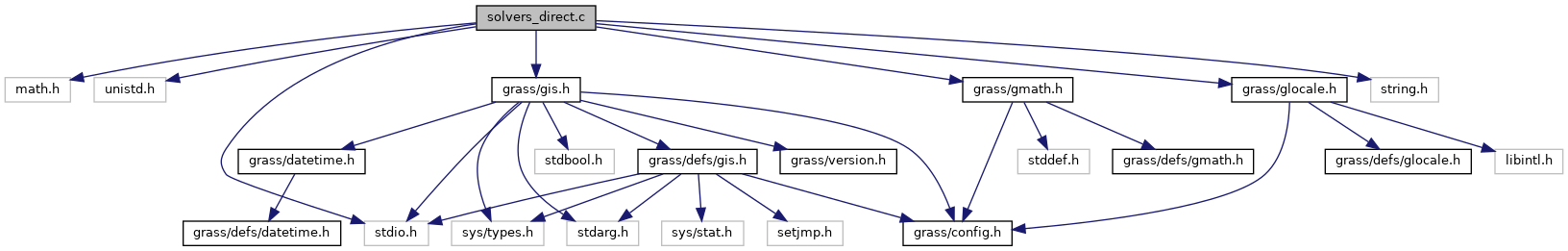GRASS GIS 7 Programmer's Manual  7.9.dev(2021)-e5379bbd7
solvers_direct.c File Reference
`#include <math.h>`
`#include <unistd.h>`
`#include <stdio.h>`
`#include <string.h>`
`#include <grass/gis.h>`
`#include <grass/gmath.h>`
`#include <grass/glocale.h>`
Include dependency graph for solvers_direct.c:Go to the source code of this file.

## Macros

#define TINY   1.0e-20

#define COMP_PIVOT   100

## Functions

int G_math_solver_gauss (double **A, double *x, double *b, int rows)
The gauss elimination solver for quardatic matrices. More...

int G_math_solver_lu (double **A, double *x, double *b, int rows)
The LU solver for quardatic matrices. More...

int G_math_solver_cholesky (double **A, double *x, double *b, int bandwidth, int rows)
The choleksy decomposition solver for quardatic, symmetric positiv definite matrices. More...

void G_math_gauss_elimination (double **A, double *b, int rows)
Gauss elimination. More...

void G_math_lu_decomposition (double **A, double *b, int rows)
lu decomposition More...

int G_math_cholesky_decomposition (double **A, int rows, int bandwidth)
cholesky decomposition for symmetric, positiv definite matrices with bandwidth optimization More...

void G_math_backward_substitution (double **A, double *x, double *b, int rows)
backward substitution More...

void G_math_forward_substitution (double **A, double *x, double *b, int rows)
forward substitution More...

## ◆ COMP_PIVOT

 #define COMP_PIVOT   100

Definition at line 28 of file solvers_direct.c.

## ◆ TINY

 #define TINY   1.0e-20

Definition at line 27 of file solvers_direct.c.

## ◆ G_math_backward_substitution()

 void G_math_backward_substitution ( double ** A, double * x, double * b, int rows )

backward substitution

Parameters
 A double ** x double * b double * rows int
Returns
void

Definition at line 289 of file solvers_direct.c.

## ◆ G_math_cholesky_decomposition()

 int G_math_cholesky_decomposition ( double ** A, int rows, int bandwidth )

cholesky decomposition for symmetric, positiv definite matrices with bandwidth optimization

The provided matrix will be overwritten with the lower and upper triangle matrix A = LL^T

Parameters
 A double ** rows int bandwidth int – the bandwidth of the matrix (0 > bandwidth <= cols)
Returns
void

Definition at line 220 of file solvers_direct.c.

## ◆ G_math_forward_substitution()

 void G_math_forward_substitution ( double ** A, double * x, double * b, int rows )

forward substitution

Parameters
 A double ** x double * b double * rows int
Returns
void

Definition at line 313 of file solvers_direct.c.

## ◆ G_math_gauss_elimination()

 void G_math_gauss_elimination ( double ** A, double * b, int rows )

Gauss elimination.

To run this solver efficiently, no pivoting is supported. The matrix will be overwritten with the decomposite form

Parameters
 A double ** b double * rows int
Returns
void

Definition at line 156 of file solvers_direct.c.

## ◆ G_math_lu_decomposition()

 void G_math_lu_decomposition ( double ** A, double * b, int rows )

lu decomposition

To run this solver efficiently, no pivoting is supported. The matrix will be overwritten with the decomposite form

Parameters
 A double ** b double * – this vector is needed if its part of the linear equation system, otherwise set it to NULL rows int
Returns
void

Definition at line 189 of file solvers_direct.c.

## ◆ G_math_solver_cholesky()

 int G_math_solver_cholesky ( double ** A, double * x, double * b, int bandwidth, int rows )

The choleksy decomposition solver for quardatic, symmetric positiv definite matrices.

This solver does not support sparse matrices The matrix A will be overwritten. The result is written to the vector x

Parameters
 A double ** x double * b double * bandwidth int – the bandwidth of the band matrix, if unsure set to rows rows int
Returns
int – 1 success

Definition at line 127 of file solvers_direct.c.

## ◆ G_math_solver_gauss()

 int G_math_solver_gauss ( double ** A, double * x, double * b, int rows )

The gauss elimination solver for quardatic matrices.

This solver does not support sparse matrices The matrix A will be overwritten. The result is written to the vector x

Parameters
 A double ** x double * b double * rows int
Returns
int – 1 success

Definition at line 43 of file solvers_direct.c.

## ◆ G_math_solver_lu()

 int G_math_solver_lu ( double ** A, double * x, double * b, int rows )

The LU solver for quardatic matrices.

This solver does not support sparse matrices The matrix A will be overwritten. The result is written to the vector x in the G_math_les structure

Parameters
 A double ** x double * b double * rows int
Returns
int – 1 success

Definition at line 67 of file solvers_direct.c.